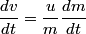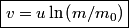## Solution to 1986 Problem 62

 It follows from the given equation that\begin{align*}\frac{dv}{dt} = \frac{u}{m} \frac{dm}{dt}\end{align*}Integrate both sides from the time when the rocket starts to$t$ gives\begin{align*}\boxed{v = u \ln \left(m/m_0\right)}\end{align*}So, answer (E) is correct.